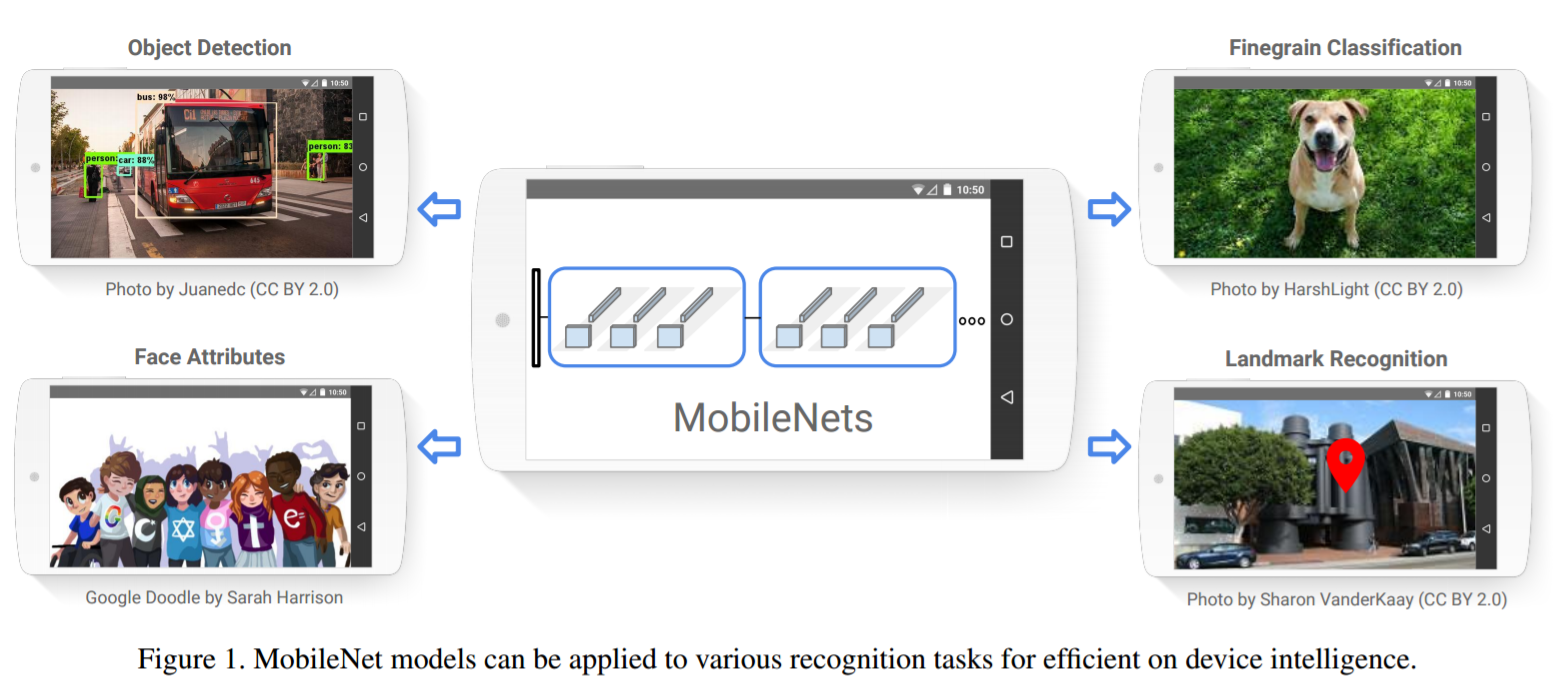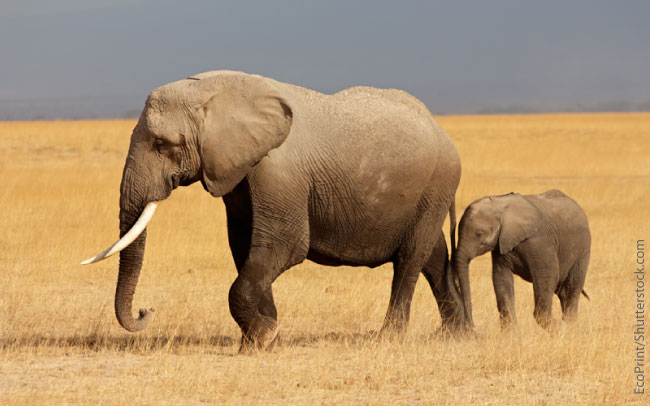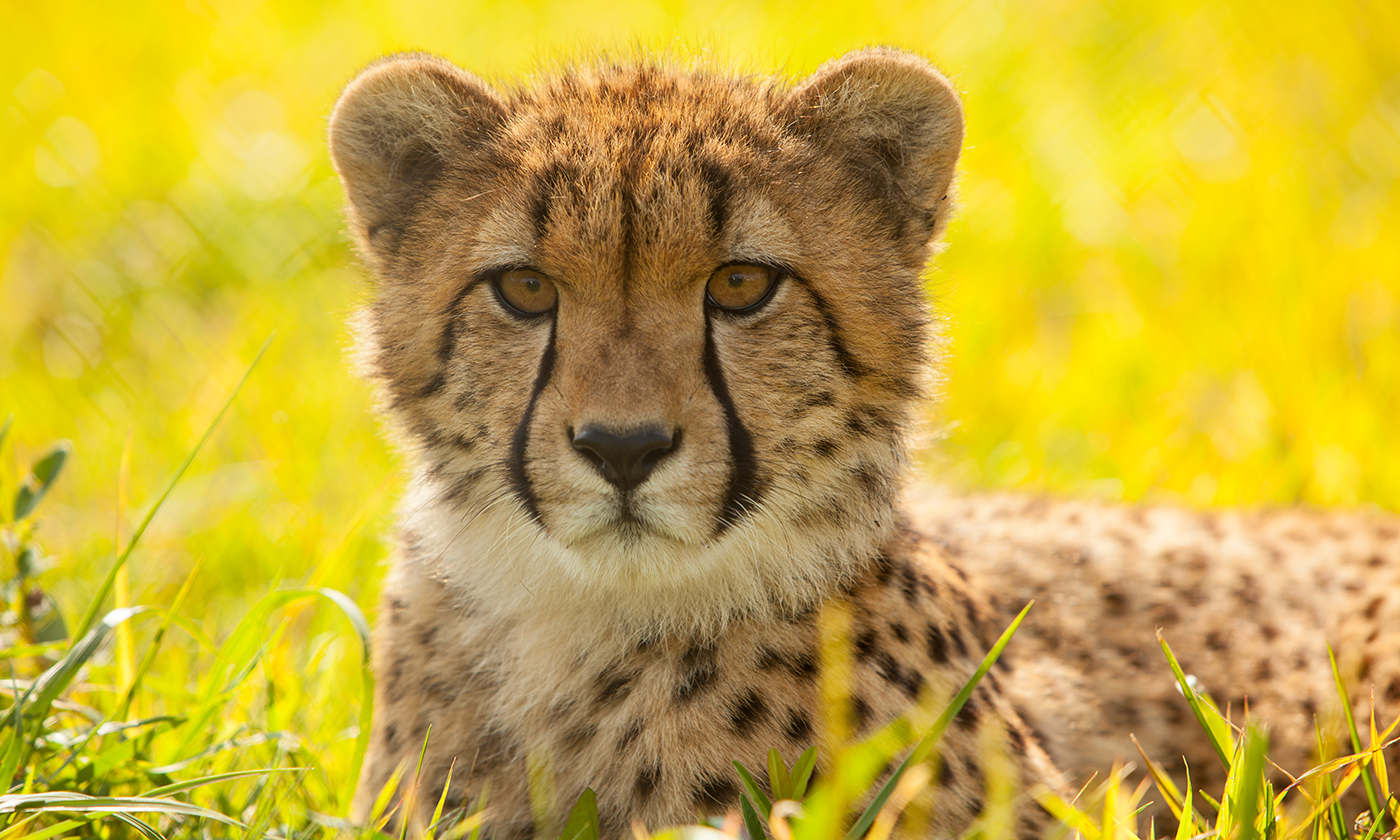# Mobile Networks (V1 and V2) in Keras

Keras implementation of the paper MobileNets: Efficient Convolutional Neural Networks for Mobile Vision Applications + ported weights.

Contains the Keras implementation of the paper MobileNetV2: Inverted Residuals and Linear Bottlenecks + ported weights.# Benefits of Mobile Nets

As explained in the paper, large neural networks can be exorbitant, both in the amount of memory they require to perform predictions, to the actual size of the model weights.

Therefore, by using Depthwise Convolutions, we can reduce a significant portion of the model size while still retaining very good performance.

# Creating MobileNets

The default MobileNet corresponds to the model pre-trained on ImageNet. It has an input shape of (224, 224, 3).

You can now create either the original version of MobileNet or the MobileNetV2 recently released using the appropriate method.

``````from mobilenets import MobileNet, MobileNetV2

# for V1
model = MobileNet()

# for V2
model = MobileNetV2()
``````

## MobileNet V1

There are two hyperparameters that you can change - `alpha` (the widening factor), and `depth_multiplier`. The ImageNet model uses the default values of 1 for both of the above.

``````from mobilenets import MobileNet

model = MobileNet(alpha=1, depth_multiplier=1)
``````

## MobileNet V2

There are three hyperparameters that you can change - `alpha` (the widening factor), `expansion_factor` (multiplier by which the inverted residual block is multiplied) and `depth_multiplier`. The ImageNet model uses the default values of 1 for `alpha` and `depth_multiplied` and a default of 6 for `expansion_factor`.

``````from mobilenets import MobileNetV2

model = MobileNetV2(alpha=1, expansion_factor=6, depth_multiplier=1)
``````

# Testing

The model can be tested by running the `predict_imagenet.py` script, using the given elephant image. It will return a top 5 prediction score, where "African Elephant" score will be around 97.9%.

 Image Predictions('African_elephant', 0.814673136), ('tusker', 0.15983042), ('Indian_elephant', 0.025479317), ('Weimaraner', 6.0817301e-06), ('bison', 3.7597524e-06)('cheetah', 0.99743026), ('leopard', 0.0010753422), ('lion', 0.00069186132), ('snow_leopard', 0.00059767498), ('lynx', 0.00012871811)

## Conversion of Tensorflow Weights

The weights were originally from https://github.com/tensorflow/models/blob/master/slim/nets/mobilenet_v1.md, which used Tensorflow checkpoints. There are scripts and some documentation for how the weights were converted in the `_weight_extraction` folder.

The weights for V2 model were originally from https://github.com/tensorflow/models/tree/master/research/slim/nets/mobilenet, which used Tensorflow checkpoints. There are scripts and some documentation for how the weights were converted in the `_weight_extraction_v2` folder.

Get A Weekly Email With Trending Projects For These Topics
No Spam. Unsubscribe easily at any time.
Python (1,126,504
Tensorflow (12,571
Neural Network (8,407
Keras (5,661
Imagenet (300
Related Projects